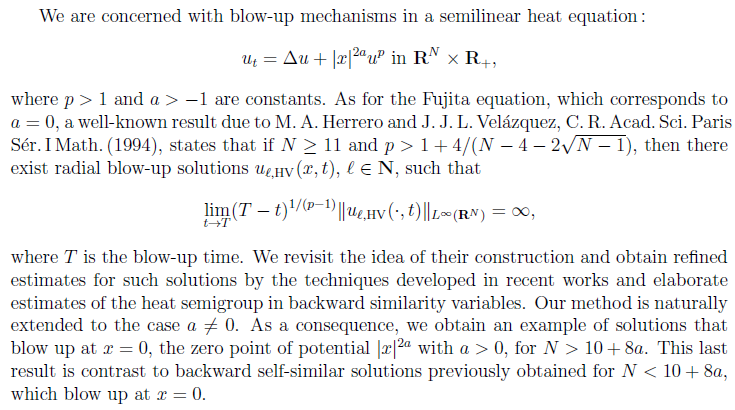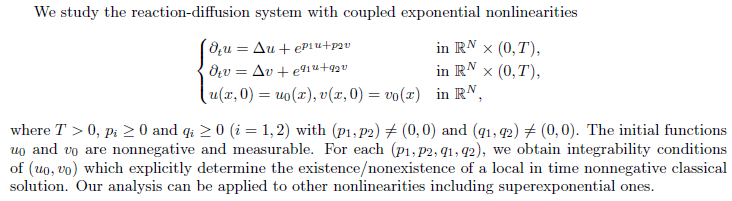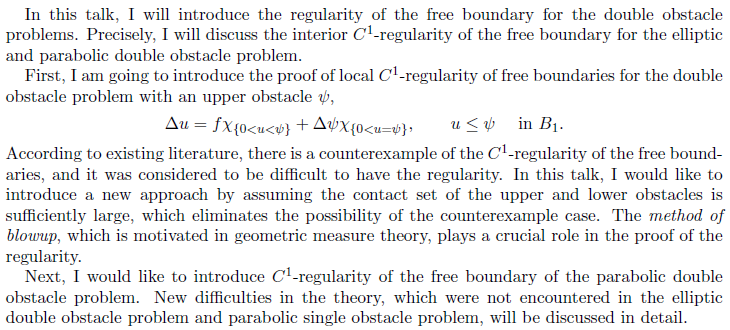ï»¿ [Math] 2020 Seoul-Tokyo Conference
 2020 Seoul-Tokyo Conference - Partial Differential Equations -   November 27 - 28, 2020          Virtual Conference
 Abstracts Home > Abstracts

Eom, Junyong (University of Tokyo)

- Title: Asymptotic expansion of ODE type solutions to a nonlinear parabolic system

- Abstract: In this talk, we investigate the large time behavior of a solution to the Cauchy problem for a nonlinear parabolic system, which behaves like ODE solution (ODE type solution). The parabolic system driven by a certain transformation caused by ODE solution has a special symmetry structure which enable us to apply known theories of higher order asymptotic expansions for scalar parabolic equations. As a result, we describe ODE type solution by use of the solutions to the heat equation and show what is distinguished the system from the scalar equation. This research is the joint work with Prof. Ishige (The University of Tokyo).

Miura, Tatsuya (Tokyo Institute of technology)

- Title: On the isoperimetric inequality and surface diffusion flow for multiply winding curves

- Abstract: In this talk we discuss dynamical stability of multiply covered circles under the surface diffusion flow. To this end we first establish a general form of the isoperimetric inequality for immersed closed curves under rotational symmetry, which would be of independent interest. We then apply it to obtaining a certain class of rotationally symmetric initial curves from which solutions to the surface diffusion flow exist globally-in-time and converge to multiply covered circles. This talk is based on a joint work with Shinya Okabe at Tohoku University.

Mitake, Hiroyoshi (University of Tokyo)

- Title: Large time behavior for a Hamilton-Jacobi equation in a critical Coagulation-Fragmentation model

- Abstract: We study the large time behavior of the sublinear viscosity solution to a singular Hamilton-Jacobi equation that appears in a critical Coagulation-Fragmentation model with multiplicative coagulation and constant fragmentation kernels. Our results include complete characterizations of stationary solutions and optimal conditions to guarantee large time convergence. In particular, we obtain convergence results under certain natural conditions on the initial data, and a nonconvergence result when such conditions fail. This is a joint work with H. V. Tran (U. Wisconsin-Madison), and T. S. Van (Carnegie Mellon University).

Mukai, Asato (University of Tokyo)

- Title: Refined construction of type II blow-up solutions for semilinear heat equations with Joseph--Lundgren supercritical nonlinearity

- Abstract:Sato, Shoichi (University of Tokyo)

- Title: Equivalence of viscosity solutions and distributional solutions for the time-fractional heat equation

- Abstract: I will talk about the equivalence of viscosity solutions and distributional solutions for the time-fractional heat equation. The definition of distributional solutions is based on the variational principle, and the definition of viscosity solution is based on the maximum value principle. Because the two theories focus on different properties of the function, it is nontrivial whether the equivalence of the two notions of weak solutions. The keys to the proof are a resolvent-type approximation of the equation and a piecewise linear approximation of the Riemann-Liouville kernel. This is joint work with Yoshikazu Giga (University of Tokyo) and Hiroyoshi Mitake (University of Tokyo).

Suzuki, Masamitsu (University of Tokyo)

- Title: Local existence and nonexistence for reaction-diffusion systems with coupled exponential nonlinearities

- Abstract:Tsuruhashi, Tomonori (University of Tokyo)

- Title: ON IRREGULAR PAIRS SATISFYING A DIVERGENCE EQUATION

- Abstract: This talk concerns a divergence equation. It is a time-independent transport equation with an external force. For a given external force, we construct an irregular pair of a scalar density and a vector field, which satisfies a divergence equation. The construction of this pair is based on the convex integration method. This shows that there exists a pair which violates the chain rule of differentiation. In contrast, a more regular pair cannot provide such a violation. In the end, we give a microscopic interpretation to these results.

Zhang, Longjie (University of Tokyo)

- Title: CURVATURE FLOW WITH DRIVING FORCE ON FIXED BOUNDARY POINTS

- Abstract: In this paper, we consider the curvature flow with driving force on fixed boundary points in the plane. We give a general local existence and uniqueness result of this problem with C^2 initial curve. For a special family of initial curves, we classify the solutions into three categories. Moreover, in each category, the asymptotic behavior is given.

Hong, Younghun (Chung-Ang University)

- Title: Quantum free energy minimizers for the gravitational Hartree equation, and their semi-classical limits

- Abstract: We consider the gravitational Hartree equation in the Heisenberg picture, describing the mean-field dynamics of a large number of bosonic stars. In this talk, we introduce the variational problem for (generalized) free energies of the Hartree equation. Then, we discuss interesting questions comparing with the one-particle Bose-Einstein condensate case as well as the Newtonian case. In addition, we present how quantum free energy minimizers are connected to their classical counterparts via the semi-classical limit. This talk is based on joint work with Woocheol Choi and Jinmyoung Seok.

Jeong, In-Jee (KIAS)

- Title: Vortex stretching and enhanced dissipation for the incompressible Navier-Stokes equations

- Abstract: Recent numerical simulations by Goto, Saito, and Kawahara shows that turbulence flows consist of a hierarchy of anti-parallel vortex tubes. Inspired by their work, we construct a sequence of smooth solutions to the 3D Navier-Stokes equations which exhibit enhanced dissipation of the kinetic energy in short timescales. This is based on a joint work with T. Yoneda.

Kim, Doheon (KIAS)

- Title: Entropy production estimate of the ES-BGK model

- Abstract: The ellipsoidal statistical BGK (ES-BGK) model is a generalized version of the BGK model where the local Maxwellian is generalized to an ellipsoidal Gaussian with a Prandtl parameter so that the model can produce the correct transport coefficient in the Navier-Stokes limit. The correct Prandtl number is obtained when the Prandtl parameter is -1/2, but various familiar structure of the original BGK model breaks down in this case. In this talk, we consider our recent result on the entropy-entropy production estimate of the ES-BGK model. This talk is based on a joint work with Myeong-Su Lee and Seok-Bae Yun.

Lee, Ki-ahm (Seoul National University)

- Title: HIGHER ORDER CONVERGENCE IN HOMOGENIZATIONS
- Abstract: In this talk, we are going to higher order convergence in Homogenization process through higher order interior and boundary correctors in various Nonlinear equations. Similar issues arises in vanishing viscous Hamiltonian Equations.

Lee, Taehun (KIAS)

- Title: Flows by powers of the Gauss curvature with a moving obstacle

- Abstract: We consider the obstacle problem for the flow by any positive power of the Gauss curvature to prevent the development of singularities in the flow. With a time-varying obstacle, we will prove the optimal C^{1,1}-regularity of solutions for any dimension and any exponent by considering a singular perturbation problem. Moreover, we will describe the long-time behavior of solutions which converges to the obstacle. This is a joint work with Ki-Ahm Lee.

Park, Jinwan (Seoul National University)

- Title: The Regularity Theory for the Double Obstacle Problem

- Abstract:Park, Jung-Tae (KIAS)

- Title: Some integrability results for porous medium type equations with measure data

- Abstract: In this talk, we consider porous medium type equations with measurable coefficients when the right-hand side is a nonnegative Radon measure with finite total mass. We discuss integrability results for the spatial gradient of a solution in the setting of the Marcinkiewicz space, under a suitable density condition of the right-hand side measure. We also introduce a notion of solution which guarantees the regularity results. This is joint work with Sukjung Hwang.